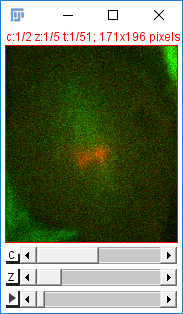# MATLAB Scripting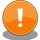Prior to MATLAB R2017b, MATLAB ships with Java 7, but ImageJ2 requires Java 8. You will need to change your MATLAB installation to use Java 8, by following the instructions for your platform: Windows, macOS or Linux.

If you run MATLAB R2017b and later, you don’t need to do this, as it ships and uses Java 8 already.

ImageJ-MATLAB is an extension which:

• Translates data between ImageJ2 images and MATLAB matrices.
• Enables execution of MATLAB scripts from inside ImageJ2’s Script Editor.
• Lets you launch ImageJ2 and interact with it from inside MATLAB.
• Allows developers to write additional plugins which extend these capabilities in new directions.

# MATLAB tutorial for ImageJ2

## Prerequisites

• Add the ImageJ-MATLAB update site. See Following an update site for more detail.
1. You go to HelpUpdate…
2. Once checking status is done, click
3. Tick “ImageJ-MATLAB” and then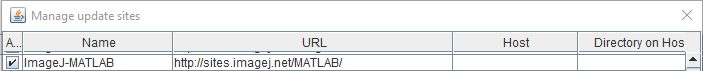4. And then click on ImageJ Updater
5. This will literally update and replace the existing, non-functional `ImageJ.m` file in the scripts folder with the genuine one.
• You will need to install your own licensed copy of MATLAB. All that ImageJ-MATLAB provides is adapters for evaluating scripts written in ImageJ2 to MATLAB, and converters between ImageJ2 and MATLAB data structures.
• Prior to MATLAB R2017b, MATLAB ships with Java 7, but ImageJ2 requires Java 8. You will need to change your MATLAB installation to use Java 8, by following the instructions for your platform: Windows, macOS or Linux. If you run MATLAB R2017b and later, you don’t need to do this, as it ships and uses Java 8 already.
• The command `version -java` will return the current Java version used by MATLAB
• If you’re new to MATLAB, first check out Mathworks’ getting started guide.
• If you’re familiar with the MATLAB language but haven’t written .m scripts before, look through the script examples.

### Memory issue

• In order to handle large images, the default Java Heap Memory size assigned won’t be enough and you may get the error `java.lang.OutOfMemoryError: Java heap space`.
• From MATLAB R2010a onward, you can increase the Jave Heap Memory size from PreferencesGeneralJava Heap Memory.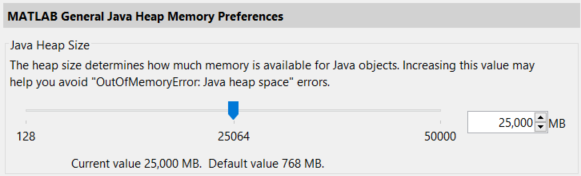• However, the maximum value allowed in the Preferences can still be too small for your purpose. In that case, you can directly edit `matlab.prf` file in the folder specified by the `prefdir<\\code> MATLAB function (eg. `C:\Users\xxxxxxx\AppData\Roaming\MathWorks\MATLAB\R2018b`). Find the parameter `JavaMemHeapMax` in the file and increase the number that follows the capital I (in MB) to increase the maximum Java heap memory size. The change will be reflected by the Preferences as above.`
``````JavaMemHeapMax=I25000
``````
• Alternatively, this can also be done by creating a `java.opts` file in the startup directory and overriding the default memory settings (see this documentation for more information). For instance, `-Xmx512m` in your `java.opts` file may be a good start point.
• For the common “Out of Memory” error, see the Troubleshooting page.

## Creating MATLAB scripts inside ImageJ2

Using the Script Editor you will be able to select MATLAB from the language menu. You can also install and run .m scripts via the standard script plugin infrastructure.

Actually running a MATLAB script from ImageJ2 is effectively like calling `eval` on the script’s contents. The script will be evaluated as such in a remote MATLAB instance (which will be launched automatically, if needed). Note that only scripts, not functions, can be evaluated in this way. See the MATLAB documentation for an explanation of these concepts.

NB: Because of the similarity to `eval`, when you need to assign character vector or string with special characters, they need to be escaped. A typical example is the handling of file/folder paths in Windows. Thus,

``````s = 'C:\Users\xxxxx\Desktop'
``````

will end up in an error in MATLAB:

``````Warning: Escaped character '\U' is not valid. See 'doc sprintf' for
supported special characters.
scijava_script523255 =
's = 'C:'
Error: Character vector is not terminated properly.
``````

You need to escape backslashes as below:

``````s = 'C:\\Users\\xxxxx\\Desktop'

s =
'C:\Users\xxxxx\Desktop'
``````

For more details, see documetation for `sprintf` and learn about Special Characters.

Options for controlling the startup of MATLAB, or killing existing MATLAB processes (e.g. if hidden) can be accessed via: EditOptionsMATLAB…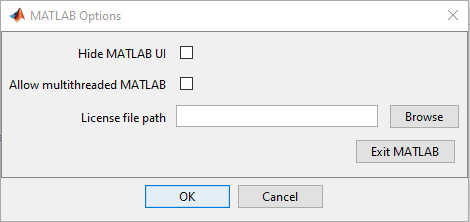Because the script is being passed from ImageJ2 to a remote MATLAB, MATLAB will not have access to ImageJ2’s classpath. Objects can be passed as variables to MATLAB (e.g. by using script parameters) but only if they are valid MATLAB classes or specially handled classes.

Scripts requiring direct access to classes from ImageJ2, or from the original ImageJ (without auto-conversion support) should launch ImageJ2 from within MATLAB.

E.g. a script using `ij.IJ` commands needs to be run from within MATLAB (or you’ll need to add Java class paths to MATLAB search path before calling `ij.IJ` commands).

For example, by default all MatlabNumericArrays will be converted to matrices within MATLAB. We also support auto-conversion of ImageJ2 `Dataset` out of the box, which can be read in by scripts using `matrix` parameters:

``````#@ matrix data
#@output net.imagej.Dataset rval

% Performs dilation with a 3x3 square,
% operating on the active dataset
% Outputs the dilated mask and the original image

rval = uint8(data); % convert to uint8
rval = mat2gray(rval); % normalize data
se = strel('square',3); % create structure to use in dilation
``````

This script will take the active `Dataset`, set it as an array variable named “data” in MATLAB, and set the matrixSum output value to the sum of the first three dimensions of the dataset.

### Global state

MATLAB retains state (e.g. declared variables) as commands are executed, and ImageJ2 makes no special effort to clean up after a script. So whether running internally or communicating externally with MATLAB, state will be available to and persist after script execution. Thus one can, for example, write scripts in ImageJ2 referencing variables declared in MATLAB, without actually initializing them in the script.

### Passing Objects

The caveat to global state is that, when running ImageJ2 externally, ImageJ2 and MATLAB run in separate JVMs. As a result, most objects can not be passed between the two. This makes `Dataset`s (and arrays) the currency that is passed between these applications.

### Return values

Most of the scripting languages supported by ImageJ2 have implicit return values. As mentioned above, ImageJ2 will only execute true scripts, which do not have return values (in the MATLAB functional sense). There is a similar concept in the `ans` variable, which automatically gets the return value of executed statements if they are not explicitly assigned. However, due to the global nature of the ImageJ-MATLAB script language, it is not necessarily clear if `ans` was set by the script or a pre-existing command. Thus the decision was made that ImageJ-MATLAB scripts will never implicitly return a value. Instead, the `#@output` annotation should always be used - even for `ans`, as shown here:

``````#@output double[] ans

% This trivial script demonstrates the use of
% the "ans" variable in the SciJava-MATLAB
% script language.

% Unassigned statements in MATLAB are automatically
% assigned to "ans". However, these scripts will not
% return "ans" unless it is explicitly requested as
% an output parameter.

0
``````

### Importing classes

To reference Java classes from MATLAB you will need to import them.Unlike the original ImageJ, ImageJ2 (and therefore Fiji) does not automatically import any classes. Consequently, scripts written for ImageJ will not run in ImageJ2 without adding the proper imports. The rationale is that the auto-import feature is not safe. What if two classes of the same name live in two different packages? Or if a new class is introduced that makes formerly unique names ambiguous? All of a sudden, all of the scripts that reference the original class no longer work. In short: auto-imports are dangerously imprecise.

When running ImageJ2 externally, MATLAB will not have ImageJ2 classes in its classpath - so they can not simply be imported. Although MATLAB does support editing its classpath this is NOT recommended, as the classes loaded by MATLAB will not be the same as those loaded in ImageJ2.

Instead, you can launch ImageJ2 inside MATLAB and have it take care of managing the class loading for you. MATLAB then supports the use of import statements to simplify class names.

## Running ImageJ2 within MATLABMATLAB versions prior to R2017b need to be tweaked to use Java 8 instead of Java 7.

The ImageJ-MATLAB update site provides an `ImageJ.m` script that will start up an ImageJ2 instance inside a running MATLAB application. Launching the script is the same as for Miji:

``````addpath '/Applications/Fiji.app/scripts' % Update for your ImageJ2 (or Fiji) installation as appropriate
ImageJ;
``````

Now, you should see a new ImageJ2 instance shows up as a window.In your MATLAB base workspace, you’ll find a variable `IJM`, which is a `net.imagej.matlab.ImageJMATLABCommands` Java object. `IJM` offers a few useful methods as below:

• `IJM.getDataset()` creates a MATLAB matrix from the active ImageJ2 image using its window title as the variable name (incompatible characters like `.` will be escaped with `_`). The MATLAB matrix is in `double` type. Although the X and Y axes are swapped, the third to fifth dimensions of the data are preserved.

• `IJM.getDatasetAs(name)` creates a MATLAB matrix from the active ImageJ2 image, and assigns it to the specified variable name `name`.

• `IJM.show(name)` takes the MATLAB matrix with the specified name `name` and displays it as an image in ImageJ2. The image is always in 32bit type. The X and Y axes are swapped. The third to fifth dimensions of the original MATLAB matrix are all piled in the fifth dimension and interpreted as time frames.

In the MATLAB command window, you’ll see something like this:

``````--------------------------------------------------------------
ImageJ-MATLAB 0.7.2: MATLAB to ImageJ Interface
--------------------------------------------------------------
JVM> Version: 1.8.0_144
JVM> Total amount of memory: 370176 Kb
JVM> Amount of free memory: 122756 Kb

-- Welcome to ImageJ-MATLAB --
ImageJ-MATLAB consists of an extensible set of commands for passing information between ImageJ and MATLAB.
See the individual sections below for a list of available commands.

https://imagej.net/scripting/matlab

--- MATLAB Command Plugins ---

-- ImageJ MATLAB commands --

Usage: IJM.[command]
help - prints a brief description of available commands
getDataset - creates a MATLAB matrix from the active ImageJ image
getDatasetAs(name) - creates a MATLAB matrix from the active ImageJ image, and assigns it to the specified variable name
show(name) - takes the MATLAB matrix with the specified name and displays it as an image

--------------------------------------------------------------
Status> ImageJ is running.
--------------------------------------------------------------
``````

The startup process automatically injects the ImageJ2 classpath into the MATLAB classpath, merging the two. At this point, you’ll have a working ImageJ2 and can now run MATLAB scripts as normal with access to the full unified classpath.

### Useful Commands

#### Show a MATLAB array `I` as an image in ImageJ2

• using `IJM`

``````IJM.show('I');
``````
• using the original ImageJ’s `ImagePlus` (see)

``````imp = copytoImagePlus(I);
imp.show();
``````
• using ImgLib2 `ArrayImg`

``````img = copytoImg(I);
net.imglib2.img.display.imagej.ImageJFunctions.show(img);
``````

#### Retrieve an image data in ImageJ as a MATLAB array `I`

• using `IJM` with the current window title as the variable name

``````IJM.getDataset();
``````
• using `IJM` with the variable name `I`

``````IJM.getDatasetAs('I');
``````
• from ImgLib2 `Img`

``````I = copytoMATLAB(img);
``````

#### Quit the ImageJ instance from MATLAB command line

`````````matlab
ij.IJ.run("Quit","");
```
``````

### Known Issues

#### Common Issues

• Although the first and second dimensions, respectively, of MATLAB array are treated as rows (Y)and columns (X) in MATLAB, the first and second dimensions of an array are treated as X and Y, respectively, in ImageJ2. The axes are transposed by `IJM.show(name)`, `IJM.getDataset()`, `IJM.getDatasetAs(name)`. (See discussion here for details)
• `IJM` object is always added to the MATLAB base workspace. In order to access `IJM` methods from within a MATLAB function, you’ll need to use `assignin`, `evalin`, and/or `global` (see here for details)
• When launching ImageJ2, you’ll see the error message below:

``````log4j:WARN No appenders could be found for logger (loci.formats.ClassList).
log4j:WARN Please initialize the log4j system properly.
``````

#### Issues specific to `IJM.show(name)`

• A multi (>2) dimensional image (with multi-channels, slices or time frames) is treated as single channel images with multiple time frames irrespective of the dimensions. For example, if a MATLAB array `A` has the size `[i,j,k]`, where `i`, `j`, and `k` correspond to the sizes in rows, columns and channels, then `IJM.show(name)` will show it as `[j,i,1,1,k]`, where X and Y axes are flipped over (see below) and channels are interpreted as frames. On the other hand, `IJM.getDataset()` and `IJM.getDatasetAs(name)` can maintain the dimensions of the image data (except the flipping over of X and Y axes).
• From GUI, you can fix this by ImageHyperstacksRe-order Hyperstack…
• For a scripting-based solution, you can run `ij.IJ.run("Re-order Hyperstack ...", "channels=[Frames (t)] slices=[Slices (z)] frames=[Channels (c)]");` in MATLAB for the example above to have dimensions `[i,j,k,1,1]` in XYCZT format. See here for details.
• When you have multiple channels, slices and frames, they’re all damped into the fifth dimension, so it takes extra effort to correct the data format.
• Also, data is always handled as 32bit (int32) per channel. Conversion to 8 bit or 16 bit images require an extra care to avoid unwanted scaling of numeric values (see here for details)

#### Solution to `IJM.show(name)` issues

• Until someone can fix `IJM.show(name)` properly, consider using `copytoImagePlus` MATLAB function instead. It solves all the issues of `IJM.show(name)` descrived above and create an ImagePlus hyperstack. See the example of a 5D Hyperstack below.
• `copytoImg` and `copytoImgPlus` MATLAB functions are also available from `Fiji.app/scripts` folder to allow you convert a MATLAB array to ImageJ2 (ImgLib2) `Img` and `ImgPlus` objects, respectively. See also this page.

### Examples

#### Accessing ImageJ Java API from MATLAB

The following Java commands work in MATLAB command window to open a sample image in ImageJ.

``````imp = ij.IJ.openImage("http://imagej.net/ij/images/boats.gif");
imp.show()
``````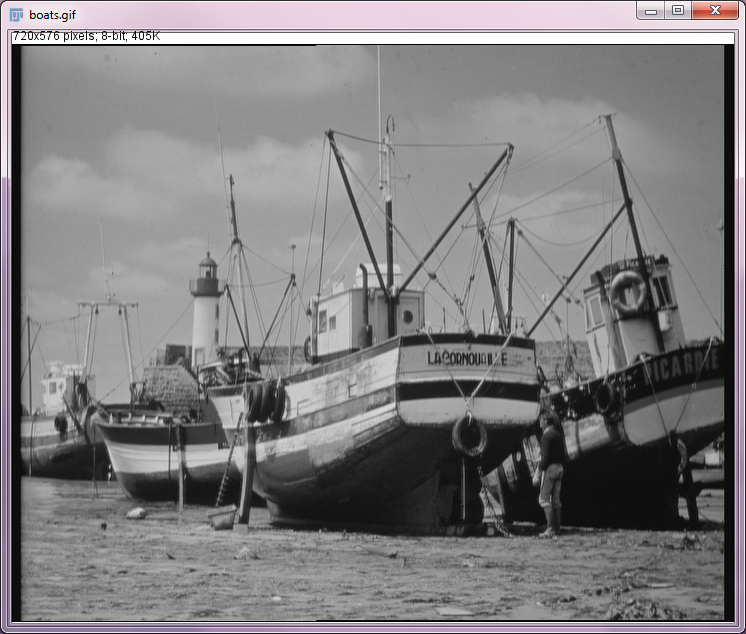#### Opening a MATLAB array as an image in ImageJ2

The following will open a MATLAB array data as an image in ImageJ2. Note that the X and Y of the image are transposed in ImageJ2 in the second image (see Limitations of `IJM.show()` above).

``````corn_gray = imread('corn.tif',3);
imshow(corn_gray); % show in MATLAB
IJM.show('corn_gray'); % show in ImageJ2
corn_gray_tp = corn_gray'; % transpose array
IJM.show('corn_gray_tp'); % show in ImageJ2 properly
``````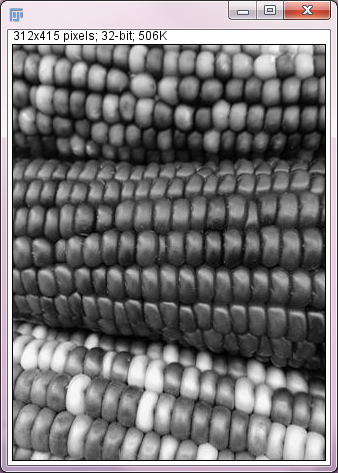A more general solution to this issue of X-Y transposition can be achieved by `permute` function of MATLAB. But please beware of memory demands by `permute`.

``````% I is a MATLAB array with 2 or more dimensions
order = [{2, 1}, num2cell(3:ndims(I))];
I2 = permute(I,[order{:}]);
IJM.show(I2) % this will show an image in proper X and Y
``````

If you wish to show an image with ImageJ from within a MATLAB function, you can achieve that by using `copytoImagePlus` as below:

``````function demofun()

imp = copytoImagePlus(corn_gray);
imp.show();

end
``````

#### Retrieving a MATLAB array data from an image in ImageJ2

Additionally, the Scripting-MATLAB library also includes an extensible MATLAB command framework. This allows for the creation of utility classes that will be automatically populated into MATLAB variables for easy access. For example, you could use ImageJ2 to open a dataset and perform thresholding (or any other processing steps), then in MATLAB use the `IJM.getDatasetAs(name)` command to set the active dataset as a MATLAB matrix variable with a specified name, for further analysis.

For example, instead of using a script as described above, we could achieve the same result by executing the following commands in the MATLAB prompt:

``````IJM.getDatasetAs('data') % import the image as a MATLAB matrix
rval = uint8(data); % convert to uint8
rval = mat2gray(rval); % normalize data
se = strel('square',3); % create structure to use in dilation
IJM.show('rval') % display the rval array in ImageJ2
``````

If you wish to assign the numeric data of an ImageJ2 image into the Workspace of a MATLAB function, rather than the base Workspace, you can achieve that by making the variable global as below:

``````function demofun()

evalin('base','global data');
evalin('base','IJM.getDatasetAs(''data'')');
global data % data is now available in the Workspace of this function
disp(size(data))

clear global data % leaving a global varibale can cause unexpected problems

end
``````

#### Opening a 2D-5D MATLAB array as a Hyperstack in ImageJ

If you use `copytoImagePlus`, you can open a 2D-5D MATLAB array as a Hyperstack with the right image type (8bit, 16bit, and 32bit for uint8, uint16, and other types of MATLAB array, respectively) in ImageJ with a simple syntax. Also, you can use `copytoImagePlus` within a MATLAB function independently of the `IJM` variable in base workspace.

``````imp = ij.IJ.openImage("http://imagej.net/ij/images/Spindly-GFP.zip");
imp.show();
IJM.getDatasetAs('I'); % I is 5-D double with the size of 171x196x2x5x51 in MATLAB
I16 = uint16(I);

IJM.show('I16') % ImagePlus, 32-bit, 171x196x1x1x510

imp4 = copytoImagePlus(I16,'XYCZT') % ImagePlus, 16-bit, 171x196x2x5x51
imp4.show();
``````

`IJM.show(name)` cannot reproduce the dimensions and the data type. Channels (C), slices (Z), and frames (T) are all treated as frames. Image type is 32 bit.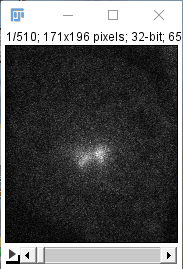`copytoImagePlus` can keep the dimensions and the data type.